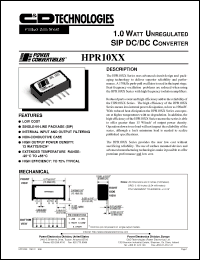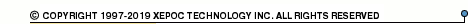More than4 307 195 components listedHPR1000 series datasheets. Manufacturer: CANDD.

 HPR1000 1.0 Watt unregulated DC/DC converter. Nom.input voltage 5Vdc, rated output voltage 5Vdc, rated output current 200mA. in 5-pin SIP package. Operational temperature range from -25°C to 65°C. Datasheet*) HPR1000H 1.0 Watt unregulated DC/DC converter. Nom.input voltage 5Vdc, rated output voltage 5Vdc, rated output current 200mA. in 5-pin SIP package. Operational temperature range from -25°C to 65°C. Datasheet*) HPR1001 1.0 Watt unregulated DC/DC converter. Nom.input voltage 5Vdc, rated output voltage 12Vdc, rated output current 83mA. in 5-pin SIP package. Operational temperature range from -25°C to 65°C. Datasheet*) HPR1001H 1.0 Watt unregulated DC/DC converter. Nom.input voltage 5Vdc, rated output voltage 12Vdc, rated output current 83mA. in 5-pin SIP package. Operational temperature range from -25°C to 65°C. Datasheet*) HPR1002 1.0 Watt unregulated DC/DC converter. Nom.input voltage 5Vdc, rated output voltage 15Vdc, rated output current 67mA. in 5-pin SIP package. Operational temperature range from -25°C to 65°C. Datasheet*) HPR1002H 1.0 Watt unregulated DC/DC converter. Nom.input voltage 5Vdc, rated output voltage 15Vdc, rated output current 67mA. in 5-pin SIP package. Operational temperature range from -25°C to 65°C. Datasheet*) HPR1003 1.0 Watt unregulated DC/DC converter. Nom.input voltage 5Vdc, rated output voltage +-5Vdc, rated output current +-100mA. in 5-pin SIP package. Operational temperature range from -25°C to 65°C. Datasheet*) HPR1003H 1.0 Watt unregulated DC/DC converter. Nom.input voltage 5Vdc, rated output voltage +-5Vdc, rated output current +-100mA. in 5-pin SIP package. Operational temperature range from -25°C to 65°C. Datasheet*) HPR1004 1.0 Watt unregulated DC/DC converter. Nom.input voltage 5Vdc, rated output voltage +-12Vdc, rated output current +-42mA. in 5-pin SIP package. Operational temperature range from -25°C to 65°C. Datasheet*) HPR1004H 1.0 Watt unregulated DC/DC converter. Nom.input voltage 5Vdc, rated output voltage +-12Vdc, rated output current +-42mA. in 5-pin SIP package. Operational temperature range from -25°C to 65°C. Datasheet*) HPR1005 1.0 Watt unregulated DC/DC converter. Nom.input voltage 5Vdc, rated output voltage +-15Vdc, rated output current +-34mA. in 5-pin SIP package. Operational temperature range from -25°C to 65°C. Datasheet*) HPR1005H 1.0 Watt unregulated DC/DC converter. Nom.input voltage 5Vdc, rated output voltage +-15Vdc, rated output current +-34mA. in 5-pin SIP package. Operational temperature range from -25°C to 65°C. Datasheet*) HPR1006 1.0 Watt unregulated DC/DC converter. Nom.input voltage 12Vdc, rated output voltage 5Vdc, rated output current 200mA. in 5-pin SIP package. Operational temperature range from -25°C to 65°C. Datasheet*) HPR1006H 1.0 Watt unregulated DC/DC converter. Nom.input voltage 12Vdc, rated output voltage 5Vdc, rated output current 200mA. in 5-pin SIP package. Operational temperature range from -25°C to 65°C. Datasheet*) HPR1007 1.0 Watt unregulated DC/DC converter. Nom.input voltage 12Vdc, rated output voltage 12Vdc, rated output current 83mA. in 5-pin SIP package. Operational temperature range from -25°C to 65°C. Datasheet*) HPR1007H 1.0 Watt unregulated DC/DC converter. Nom.input voltage 12Vdc, rated output voltage 12Vdc, rated output current 83mA. in 5-pin SIP package. Operational temperature range from -25°C to 65°C. Datasheet*) HPR1008 1.0 Watt unregulated DC/DC converter. Nom.input voltage 12Vdc, rated output voltage 15Vdc, rated output current 67mA. in 5-pin SIP package. Operational temperature range from -25°C to 65°C. Datasheet*) HPR1008H 1.0 Watt unregulated DC/DC converter. Nom.input voltage 12Vdc, rated output voltage 15Vdc, rated output current 67mA. in 5-pin SIP package. Operational temperature range from -25°C to 65°C. Datasheet*) HPR1009 1.0 Watt unregulated DC/DC converter. Nom.input voltage 12Vdc, rated output voltage +-5Vdc, rated output current +-100mA. in 5-pin SIP package. Operational temperature range from -25°C to 65°C. Datasheet*) HPR1009H 1.0 Watt unregulated DC/DC converter. Nom.input voltage 12Vdc, rated output voltage +-5Vdc, rated output current +-100mA. in 5-pin SIP package. Operational temperature range from -25°C to 65°C. Datasheet*) HPR1010 1.0 Watt unregulated DC/DC converter. Nom.input voltage 12Vdc, rated output voltage +-12Vdc, rated output current +-42mA. in 5-pin SIP package. Operational temperature range from -25°C to 65°C. Datasheet*) HPR1010H 1.0 Watt unregulated DC/DC converter. Nom.input voltage 12Vdc, rated output voltage +-12Vdc, rated output current +-42mA. in 5-pin SIP package. Operational temperature range from -25°C to 65°C. Datasheet*) HPR1011 1.0 Watt unregulated DC/DC converter. Nom.input voltage 12Vdc, rated output voltage +-15Vdc, rated output current +-34mA. in 5-pin SIP package. Operational temperature range from -25°C to 65°C. Datasheet*) HPR1011H 1.0 Watt unregulated DC/DC converter. Nom.input voltage 12Vdc, rated output voltage +-15Vdc, rated output current +-34mA. in 5-pin SIP package. Operational temperature range from -25°C to 65°C. Datasheet*) HPR1012 1.0 Watt unregulated DC/DC converter. Nom.input voltage 15Vdc, rated output voltage 5Vdc, rated output current 200mA. in 5-pin SIP package. Operational temperature range from -25°C to 65°C. Datasheet*) HPR1012H 1.0 Watt unregulated DC/DC converter. Nom.input voltage 15Vdc, rated output voltage 5Vdc, rated output current 200mA. in 5-pin SIP package. Operational temperature range from -25°C to 65°C. Datasheet*) HPR1013 1.0 Watt unregulated DC/DC converter. Nom.input voltage 15Vdc, rated output voltage 12Vdc, rated output current 83mA. in 5-pin SIP package. Operational temperature range from -25°C to 65°C. Datasheet*) HPR1013H 1.0 Watt unregulated DC/DC converter. Nom.input voltage 15Vdc, rated output voltage 12Vdc, rated output current 83mA. in 5-pin SIP package. Operational temperature range from -25°C to 65°C. Datasheet*) HPR1014 1.0 Watt unregulated DC/DC converter. Nom.input voltage 15Vdc, rated output voltage 15Vdc, rated output current 67mA. in 5-pin SIP package. Operational temperature range from -25°C to 65°C. Datasheet*) HPR1014H 1.0 Watt unregulated DC/DC converter. Nom.input voltage 15Vdc, rated output voltage 15Vdc, rated output current 67mA. in 5-pin SIP package. Operational temperature range from -25°C to 65°C. Datasheet*) HPR1016 1.0 Watt unregulated DC/DC converter. Nom.input voltage 15Vdc, rated output voltage +-12Vdc, rated output current +-42mA. in 5-pin SIP package. Operational temperature range from -25°C to 65°C. Datasheet*) HPR1016H 1.0 Watt unregulated DC/DC converter. Nom.input voltage 15Vdc, rated output voltage +-12Vdc, rated output current +-42mA. in 5-pin SIP package. Operational temperature range from -25°C to 65°C. Datasheet*) HPR1017 1.0 Watt unregulated DC/DC converter. Nom.input voltage 15Vdc, rated output voltage +-15Vdc, rated output current +-34mA. in 5-pin SIP package. Operational temperature range from -25°C to 65°C. Datasheet*) HPR1017H 1.0 Watt unregulated DC/DC converter. Nom.input voltage 15Vdc, rated output voltage +-15Vdc, rated output current +-34mA. in 5-pin SIP package. Operational temperature range from -25°C to 65°C. Datasheet*) HPR1018 1.0 Watt unregulated DC/DC converter. Nom.input voltage 24Vdc, rated output voltage 5Vdc, rated output current 200mA. in 5-pin SIP package. Operational temperature range from -25°C to 65°C. Datasheet*) HPR1018H 1.0 Watt unregulated DC/DC converter. Nom.input voltage 24Vdc, rated output voltage 5Vdc, rated output current 200mA. in 5-pin SIP package. Operational temperature range from -25°C to 65°C. Datasheet*) HPR1019 1.0 Watt unregulated DC/DC converter. Nom.input voltage 24Vdc, rated output voltage 12Vdc, rated output current 83mA. in 5-pin SIP package. Operational temperature range from -25°C to 65°C. Datasheet*) HPR1019H 1.0 Watt unregulated DC/DC converter. Nom.input voltage 24Vdc, rated output voltage 12Vdc, rated output current 83mA. in 5-pin SIP package. Operational temperature range from -25°C to 65°C. Datasheet*) HPR1020 1.0 Watt unregulated DC/DC converter. Nom.input voltage 24Vdc, rated output voltage 15Vdc, rated output current 67mA. in 5-pin SIP package. Operational temperature range from -25°C to 65°C. Datasheet*) HPR1020H 1.0 Watt unregulated DC/DC converter. Nom.input voltage 24Vdc, rated output voltage 15Vdc, rated output current 67mA. in 5-pin SIP package. Operational temperature range from -25°C to 65°C. Datasheet*) HPR1021 1.0 Watt unregulated DC/DC converter. Nom.input voltage 24Vdc, rated output voltage +-5Vdc, rated output current +-100mA. in 5-pin SIP package. Operational temperature range from -25°C to 65°C. Datasheet*) HPR1021H 1.0 Watt unregulated DC/DC converter. Nom.input voltage 24Vdc, rated output voltage +-5Vdc, rated output current +-100mA. in 5-pin SIP package. Operational temperature range from -25°C to 65°C. Datasheet*) HPR1022 1.0 Watt unregulated DC/DC converter. Nom.input voltage 24Vdc, rated output voltage +-12Vdc, rated output current +-42mA. in 5-pin SIP package. Operational temperature range from -25°C to 65°C. Datasheet*) HPR1022H 1.0 Watt unregulated DC/DC converter. Nom.input voltage 24Vdc, rated output voltage +-12Vdc, rated output current +-42mA. in 5-pin SIP package. Operational temperature range from -25°C to 65°C. Datasheet*) HPR1023 1.0 Watt unregulated DC/DC converter. Nom.input voltage 24Vdc, rated output voltage +-15Vdc, rated output current +-34mA. in 5-pin SIP package. Operational temperature range from -25°C to 65°C. Datasheet*) HPR1023H 1.0 Watt unregulated DC/DC converter. Nom.input voltage 24Vdc, rated output voltage +-15Vdc, rated output current +-34mA. in 5-pin SIP package. Operational temperature range from -25°C to 65°C. Datasheet*)